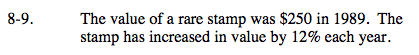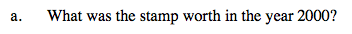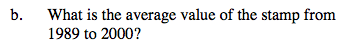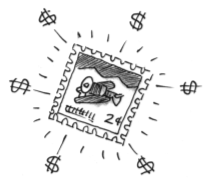### Home > CALC > Chapter 8 > Lesson 8.1.1 > Problem8-9

8-9.
1. The value of a rare stamp was $250 in 1989. The stamp has increased in value by 12% each year. Homework Help ✎ 1. What was the stamp worth in the year 2000? 2. What is the average value of the stamp from 1989 to 2000?The stamp is 112% of its value from the previous year.This will happen for 11 years in a row. 250·1.1211 =$869.64If f(t) represents the value of the stamp as a function of time (the equation you used to solve part (a)):